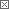Roger's Equations Blog### Roger's Equations

This blog is all about science and technology (with occasional math thrown in for fun). The goal of this blog is to try and pass on the sense of excitement and wonder I feel when I read about these topics. I hope you enjoy the posts.

 Previous in Blog: Phi - The Golden Ratio Part I Next in Blog: The Chemistry of DNA# Phi - The Golden Ratio Part II

Posted February 29, 2008 12:00 AM by Bayes

Last entry I derived Φ (pronounced "Fi" by some and "Fee" by others) by cutting a line in such a way that the ratio between the two new line segments created after the cut were the same as the ratio between the larger line segment created after the cut and the original line. I showed that when such constraints as define Φ above are imposed, there are two possible analytical solutions,

Φ=1.61803398874989.....
Φ= - 0.618033988749894....

I indicated that the first value was traditionally taken to be Φ. The second value, although a perfectly reasonable analytical solution, as a negative solution implies a negative length of one of the line segments involved (remember, Phi represents the ratio of lengths), which is not allowed.

So

Φ=1.61803398874989.....

Now what?

Properties of Phi

Recurrence Relation

The inverse of Φ is Φ - 1. (1/Φ = Φ-1)

The square of Φ is Φ + 1. (Φ2 = Φ + 1)

These two expressions are actually two examples of a more general property of Φ,notice if n=1

Φ= Φ0 + Φ-1= 1 + 1/Φ, which can be rewritten as the first equation above,
1/Φ = Φ-1

notice if n=2

Φ2= Φ1 + Φ0= Φ + 1, which is the second equation above.

Continuing Fractions

Φ can be expressed as the continuing fractions:

Φ=orFibonacci Series

The ratio of successive terms in the Fibonacci Series approaches Φ. The Fibonacci Series is:

F=0, 1, 1, 2, 3, 5, 8, 13, 21, 34, 55, 89, 144, 233, 377, 610, 987, ...

and can be expressed in terms of Φ:Noting that, as I said earlier, the ratio of respective terms of this series approaches Φ:so 2/1=2, 3/2=1.5, 5/3=1.6666, 8/5=1.6, 13/8=1.625, 21/13=1.654, 34/21=1.619, etc.

It's possible to calculate powers of Φ with Fibonacci Series terms.

Φn=F(n-1) + F(n)Φ

where F(n) is the nth term of the Fibonacci series. For example:

Φ5=3+5Φ
or
Φ12=89+144Φ

Imaginary Numbers

A neat expression that involves Φ and i is,

Sin(i lnΦ)= (1/2) i

That's it for this entry. If there is a topic you are interested in feel free to email me and I'll try to get to it.

Thanks to the following sources:

http://www.albany.edu/~rp858838

Interested in this topic? By joining CR4 you can "subscribe" to